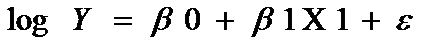# Non linear regression analysis in STATA and its interpretation

By Indra Giri and Priya Chetty on February 7, 2017

In the previous article on Linear Regression using STATA, a simple linear regression model was used to test the hypothesis. However the linear regression will not be effective if the relation between the dependent and independent variable is non linear. The non linear regression is used more in the real life as compared to the linear regression.

## Log-transformation for non linear regression

The main purpose of logarithmic transformations is to handle situations when there is non-linear relationship between independent and dependent variables. Using the logarithmic model for one or more variables will make effective non-linear relationship and also preserve the linear model. These models also are used to analyse variables in order to transform a highly skewed model into a normal one. There are three different ways to incorporate log in the regression model.

#### Linear – log regression model

In the linear log regression analysis the independent variable is in log form whereas the dependent variable is kept normal. The linear log regression analysis can be written as:In this case the independent variable (X1) is transformed into log. One can transform the normal variable into log form using the following command:

`gen lnX = log(X)  (if you want to create new variable with log transformation)  `
`replace X = log(X)     (if you want to replace the variable with its log form)`

In case of linear log model the coefficient can be interpreted as follows:

If the independent variable is increased by 1% then the expected change in dependent variable is (β/100) units, holding all other things constant.

For example the linear log regression analysis was performed using the same data set in previous articles. Here price is dependent variable and lnmpg is the independent variable. The independent variable lnmpg is the log transformation of the mpg. The coefficient of lnmpg -5992.728 can be interpreted as follows:

With 1% increase in mpg the price of auto declines by (5992.72/100)  or 59.93 units, holding all other factors constant.

Interpretation of all other components in the above table is similar to the linear regression, explained in previous article.

#### Log- linear regression model

As the name suggest in log linear regression model the dependent variable is in log form instead of the independent variable. The regression equation of the log-linear regression is as follows:The dependent variable can also be transformed into log form using the similar command given in the previous section.

The coefficient of independent variable in case of log-linear regression can be interpreted as:

If independent variable is increased by 1 unit, the dependent variable is expected to increase by (β*100)% holding all things constant.

Using the same data set, the log linear regression has been performed. In this case lnprice is the dependent variable which is the log transformation of price and independent variable mpg is in its normal form.

The coefficient of mpg can be interpreted as:

If mpg is increased by one unit the price is expected to decrease by 3.32 %, holding all other factors constant.

#### Log-log model

In log-log model both the dependent and the independent variables are in log form. The regression equation of log-log regression is as follows:The coefficient of the log log model can be interpreted as:

If independent variable increase by 1% the dependent variable will increase/ decrease by β %, holding all other factors constant.